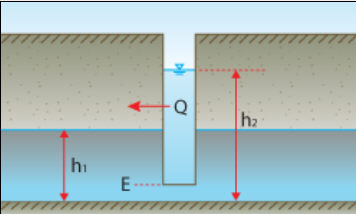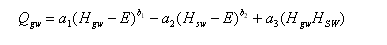#INFOSWMM

# EPA #SWMM’s Groundwater Equation

EPA #SWMM’s Groundwater EquationIntroduction: H2OCalc is a hydrology and hydraulics calculator sold by Innovyze that has many of the equations and calculation methods used in InfoSWMM, ICM and SWMM5.

The dialog box for EPA SWMM’s aquifer production equation is shown below.Input for EPA SWMM’s aquifer production equation:

Groundwater Flow – Select EPA SWMM’s aquifer production equation.

Hydraulic Conductivity – A proportionality constant that describes the ease with which water can move through pore spaces of the aquifer. It is an output if selected as a solving target.

Groundwater Table Elevation – Elevation of the groundwater table.

Water Elevation in the Node – Elevation of surface water at the receiving node.

Threshold Elevation – Threshold groundwater table elevation or elevation of node invert.

a1, a2, b1, b2, and a3 – coefficients that appear in the following equation that computes groundwater flow as a function of groundwater and surface water heads.Where: Qgw = Groundwater flow (cfs/acre, cms/per hectare)

Hgw = Elevation of groundwater table (ft, m)

Hsw = Elevation of surface water at receiving node (ft, m)

E = Threshold groundwater table elevation or elevation of node invert (ft, m).

Output for EPA SWMM’s aquifer production equation:

Discharge – Flow rate per unit width of the aquifer.